﻿ Kinetics of Leaching of Iron Oxide in Clay in Oxalic Acid and in Hydrochloric Acid Solutions

Kinetics of Leaching of Iron Oxide in Clay in Oxalic Acid and in Hydrochloric Acid Solutions

U K Sultana, Fahmida Gulshan, A S W KurnyOPEN ACCESSPEER-REVIEWED

Kinetics of Leaching of Iron Oxide in Clay in Oxalic Acid and in Hydrochloric Acid Solutions

U K Sultana1, Fahmida Gulshan1, A S W Kurny1,1Department of Materials and Metallurgical Engineering, Bangladesh University of Engineering and Technology, Dhaka, Bangladesh

Abstract

Iron oxide in samples of clay containing 8.15% iron oxide was leached in aqueous oxalic acid and hydrochloric acid solutions. Leaching experiments were conducted in the temperature range of 40–80°C for times up to 90 minutes in 0.2 M to 2 M acid solutions. The mixed kinetic mechanism seemed to be the most appropriate model to fit the kinetic data of leaching in oxalic acid while product layer diffusion controlled reaction model seemed to be the most appropriate one for leaching in hydrochloric acid. The Arrhenius activation energy for leaching in oxalic acid was found to be 41.035 kJ/mole while that for hydrochloric acid was 50.82 kJ/mole.

At a glance: Figures

1234
Prev Next

• Sultana, U K, Fahmida Gulshan, and A S W Kurny. "Kinetics of Leaching of Iron Oxide in Clay in Oxalic Acid and in Hydrochloric Acid Solutions." Materials Science and Metallurgy Engineering 2.1 (2014): 5-10.
• Sultana, U. K. , Gulshan, F. , & Kurny, A. S. W. (2014). Kinetics of Leaching of Iron Oxide in Clay in Oxalic Acid and in Hydrochloric Acid Solutions. Materials Science and Metallurgy Engineering, 2(1), 5-10.
• Sultana, U K, Fahmida Gulshan, and A S W Kurny. "Kinetics of Leaching of Iron Oxide in Clay in Oxalic Acid and in Hydrochloric Acid Solutions." Materials Science and Metallurgy Engineering 2, no. 1 (2014): 5-10.

 Import into BibTeX Import into EndNote Import into RefMan Import into RefWorks

1. Introduction

The presence of impurities, particularly iron bearing materials, impairs the characteristics of industrial raw materials such as quartz, clay and affects their utility for various applications . Traditionally iron is removed by physical separation techniques, but acid washing is gaining wide acceptance [2, 3, 4]. Recent studies have shown that even traces of iron can be removed by acid washing .

Both inorganic and organic acids have been used for acid washing. Results of investigations on leaching of iron oxides in clays in oxalic acid solutions dominate the published literature. Chiaraizia and Horwitz  studied dissolution of goethite in several organic acids in the presence of reducing agents. Panias et at  examined the mechanisms of dissolution of iron oxides in aqueous oxalic acid solution. Lee et al.  worked on the optimization of pH level and pH control during leaching in oxalic acid solution. Ambikadevi and Lalithambika  tested several organic acids and concluded that oxalic acid is most efficient to dissolve iron oxide from ceramic minerals. The kinetics of leaching of iron in diasporic bauxite  and iron ore  in hydrochloric acid has also been investigated. Sidhu et al  investigated the dissolution of synthesized iron oxides and oxyhydroxides in hydrochloric and perchloric acids. On the other hand little information on leaching of iron oxides contained in clays is available in published literature. Moreover a study of leaching of iron oxides in the same sample of clay in an organic acid and an inorganic acid may be useful in the process of selection of proper solution for washing of potential ceramic raw materials.

For kinetic studies the progressive conversion model and the shrinking unreacted core models are the two simple models generally considered for the non-catalytic reaction of particles with the surrounding fluids. In the progressive conversion model reaction occurs throughout the particles at different rates at different locations. The solid reactant is converted continuously and progressively throughout the particle. This is unrealistic in maximum leaching reactions. Hence shrinking core model is preferred for solid spherical particle reaction kinetics. Liquid film diffusion controlled process, product layer diffusion controlled process and chemical reaction controlled process, are the three models generally considered for the preliminary selection of the kinetic model. With shrinking cores, the kinetic data may also fit a mixed kinetic mechanism . Generally the result of the leaching experiments is matched with a kinetic model and data from the selected model is used to determine activation energy .

The primary aim of the present investigation was to determine the comparative effectiveness for the removal of iron in oxalic acid as well as in hydrochloric acid solution from a clay sample collected from Narsingdi (near Dhaka) in Bangladesh. The mechanism of the reaction was identified and the activation energy was determined.

2. Experimental Procedure

2.1. Material Composition

The sample of clay under investigation was collected from Narsingdi (near Dhaka). The sample, as determined by x-ray fluorescence spectroscopy, contained 27.5% of Alumina, 59.5% of silica and 8.15% of iron oxide.

2.2. Leaching Procedure

Leaching was carried out in a three necked round bottom flask fitted with a reflux condenser and a mechanical stirrer. The flask was placed on a thermostatically controlled heating mantle. Temperature of the solution was controlled to specified values and was continuously monitored by a thermometer. Samples of clay weighing 6 gm were taken in 400 ml of leaching solution. The optimum concentration of both the acids were determined by leaching in 0.2 M, 0.4 M, 0.6 M, 0.8 M, 1.0 M, 1.2 M, 1.4 M, 1.6 M, 1.8 M and 2 M acid solutions. The subsequent leaching was performed in the optimised acid concentrations at temperatures of 40, 60 and 80°C and for 1, 2, 4, 5, 10, 20, 30, 45, 60, 90 minutes at each temperature. A constant stirring speed of 600 rpm was maintained during all leaching experiments. At the end of leaching for a specified period of time at a specified temperature 5 ml of leaching solution was taken out of the round bottom flask by a pipette. The collected sample of leach liquor was cooled, filtered and used for iron estimation.

2.3. Iron Estimation Procedure

The analysis of iron was performed a in Shimadzu UV-vis Spectrophotometer using standard procedures . Tartaric acid, thyoglycholic acid, nitric acid and ammonia solution were used as complexing agents. The details of the procedure is shown in Figure 1.

Table 1. composition of the clayDownload asPowerPoint Slide

Veiw figureView current table in a new window

3. Results and Discussion

3.1. Compositional Analysis of the Clay

The composition of the clay, as determined by x-ray fluorescence spectroscopy, is shown in Table 1.

3.2. Effect of Acid Concentration

The extent of dissolution of iron as a function of concentration of oxalic acid and hydrochloric acid are shown in Figure 2. It is evident that the dissolution of iron is directly affected by concentration of acid in leach liquor. The higher the concentration of acids, the higher is the extent of dissolution of iron oxide. The increase in the extent of removal of iron is negligible at concentrations higher than 1.8 M for oxalic acid and 1.6 M for hydrochloric acid.

3.3. Effect of Time and Temperature

Figure 3 and Figure 4 show the effect of time and temperature on the rate of leaching of iron oxide in the clay sample in 1.8 M oxalic acid and in 1.6 M hydrochloric acid. It can be seen that during the initial period of leaching at all temperatures under investigation, the rate of leaching was rather fast. However, the curves became almost flat within a very short period of time, after which the increase in the extent of removal of iron was negligible. It can also be seen that the rate of leaching was faster at higher temperatures.

3.4. Identification of Reaction Mechanism

The particles were assumed to be perfectly homogenous spherical solid phases and the shrinking core model was selected to analyze the rate of dissolution of iron. Such plots are quite extensively used in kinetic studies . The kinetic relationships are expressed in terms of the fraction of reacted particle X, with time. Three established models i.e. chemical reaction controlled process, liquid film diffusion controlled process, product layer diffusion controlled process and also a mixed kinetic mechanism were considered for initial selection of the reaction mechanism. The equations for these models are as follows:

1. Chemical reaction controlled process: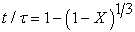(1)

2. Liquid film diffusion controlled process: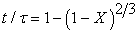(2)

3. Product layer diffusion controlled process: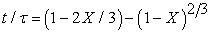(3)

4. Mixed controlled process: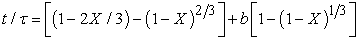(4)

where,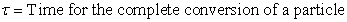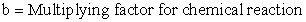To test if the process is chemical reaction controlled, time for different fractions of reacted particle of iron were calculated from Figure 3 and Figure 4 at 40°C, 60°C and 80°C. Data was then plotted according to equation 1 in Figure 5 and Figure 6 respectively. The correlation coefficient (R2) values are also shown in the figure. It can be seen that the correlation is not high enough at all temperatures to suggest that the dissolution of iron oxide in the clay sample is a chemical reaction controlled process.

Figure 5. Plot of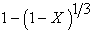vs time at various temperatures for Iron dissolution in Oxalic acid
Figure 6. Plot ofvs time at various temperatures for Iron dissolution in HCl
Figure 7. Plot ofvs time at different temperatures for iron dissolution in oxalic acid
Figure 8. Plot of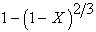vs time at different temperatures for iron dissolution in HCl

The plot ofversus time (Equation 2) at different temperatures is shown in Figure 7 and Figure 8. This equation was considered to test if the process is a liquid film diffusion controlled process. It can be seen from both figures that the correlation factor is low and the lines do not pass through the zero point. The model cannot, therefore, be selected for dissolution of iron in the present system.

To test if the process is product layer diffusion controlled process, the right hand side of Equation 3 was plotted against time for different temperatures (Figure 9 and Figure 10). It can be seen that the correlation factor is not satisfactory so that the product layer diffusion controlled process could be selected as the model for leaching in oxalic acid (Figure 9). However, the plot for leaching in hydrochloric acid (Figure 10) yielded a very high correlation at all temperatures under investigation. Moreover, the plotted lines (Figure 10) passed through the zero point. Therefore, the data of leaching in hydrochloric acid solution could be correlated to a product layer diffusion controlled process.

Figure 9. Plot of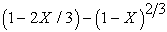vs time at different temperatures for dissolution in oxalic acid
Figure 10. plot ofversus time at different temperatures for iron dissolution in HCl

Finally, the leaching reaction in oxalic acid was assumed to follow a mixed kinetic mechanism, where the overall rate–controlling factor is a combination of diffusion controlled process and chemical reaction. The change in proportion of dominating reaction was done by setting “b” in equation (4) between 0 and 1. The values of “b” for each reaction were determined graphically by plotting the experimental data according to equation (4) for different values of “b”. The value that produced the best liners fit with the smallest 0-intercept was the one used to calculate the rate constant.

“b” is a direct function of chemical reaction and according to this mixed kinetic mechanism when “b” is very small or equal to zero, the reaction at the particle surface does not control the kinetics of dissolution and diffusion process becomes the rate controlling factor. When “b” equals 0 equation (4) becomes:The small value of “b” obtained (i.e. 0.08) suggest that the product layer diffusion controlled process was the most dominating reaction. The graphs (Figure 11) produced straight lines and passing through the zero and the correlation factor is very high compared to other mechanisms, hence the data may be assumed to fit the mixed kinetics reaction model.

Figure 11. Plot ofvs. time at different temperatures for iron dissolution in Oxalic acid
3.5. Evaluation of Activation Energy

Both the conventional integral approach and differential approach were used to evaluate the activation energy of the leaching reaction.

3.6. Integral Approach

In the integral form, t/τ versus time (t) was plotted. The reaction rate constants, k, at various temperatures of leaching were obtained from linearised plots (Figure 10 and Figure 11). The rate constant values were then plotted against temperature according to the Arrhenius type equation in Figure 12 for oxalic acid and hydrochloric acid. The plots of ln K vs. 1/T were straight lines and the slopes of the straight lines were equal to -E/R.

The apparent value of activation energy for the dissolution of iron in oxalic acid was estimated as 41.035 kJ/mole while for hydrochloric acid the value of activation energy was estimated to be 50.82 kJ/mole (Figure 12).

4. Conclusions

The following conclusions can be drawn from the study:

Ÿ  The optimum acid concentrations and temperatures were estimated to be 1.8 M and 80°C for leaching in oxalic acid and 1.6 M and 80°C for leaching in hydrochloric acid.

Ÿ  The kinetic data for iron dissolution in 1.8 M oxalic acid fits the mixed kinetic mechanism and the small value (0.08) for “b” suggests that diffusion controlled process is the dominating reaction. The kinetic data of iron dissolution in 1.6 M hydrochloric acid fits the product layer diffusion controlled model.

Ÿ  The apparent activation energy evaluated from the experimental data is 41.03458 kJ/mole for leaching in oxalic acid and 50.82 kJ/mole for leaching in hydrochloric acid.

Acknowledgement

The authors are grateful to the Project Director, Development of Materials for Tools and Biometallic Implants, Bangladesh Council for Scientific and Industrial Research for permission to use his facilities and to the authorities of Bangladesh University of Engineering and Technology, Dhaka for providing the financial support for this study.

References

  J. A. Lori, A.O.Lawal and E.J. Ekanem, Characterization and optimisation of deferration of Kankara clay, 2007. ARPN Journal of Engineering and Applied Sciences, 2 (2005) 60-72.In article  S. C. Baral, S. R. Das, S. N. Ghosh. Physico-chemical characterization of Maghalaya clays for use in ceramics. Transactions of Indian Ceramic Society, Vol. 52 (1993) 176-182.In article  S K Mukherji, B B Machhoya, A. K. Chakraborty, T K Dan. A study on the beneficiation of some crude china clays of Gujarat, Research and Industry, 38 (1993) 254-259.In article  F. Veglio, B. Passariello, M. Barbaro, P. Plescia, A.M. Marabini, Drum leaching tests in iron removal from quartz using oxalic and sulphuric acids, Int. J. Miner. Process, 54 (1998) 183-200.In article CrossRef  Suong Oh Lee, Tam Tran, Yi Yong Park, Seong Jun kim, MyongJun Kim. 2006. Study on the kinetics of Iron oxide leaching by oxalic acid, Int. J. Miner. Process, 80 (2006) 144-152.In article CrossRef  R. Chiarizia, E.P. Horwitz, New formulations for iron oxides dissolution, Hydrometallurgy 27 (1991) 339-360.In article CrossRef  D. Panias, M. Taxiarchou, I. Paspaliaris, A. Kontopoulos, Mechanisms of dissolution of Iron Oxides in aqueous oxalic acid solution, Hydrometallurgy, 42 (1996) 257-265.In article CrossRef  Sung Oh Lee, Tam Tran, Byoung Hi Jung, Seong Jun Kim, Myong Jun Kim, Dissolution of Iron oxide using oxalic acid. Hydrometallurgy, 87 (2007) 91-99.In article CrossRef  V.R. Ambikadevi, M. Lalithambika, Effect of organic acids on ferric iron removal from iron stained kaolinite. Applied Clay Science 16 (2000) 133-145.In article CrossRef  Gulsemin Gulfen, Mustafa Gulfen, Ali Osman Aydin, Dissolution Kinetics of iron from diasporic bauxite in hydrochloric acid solution, Indian Journal of Chemical Technology, 13 (2006) 386-390.In article  Alafara, A. Baba; Adekola, F A; Folashade, A. O., Quantitative Leaching of a Nigerian Iron Ore in Hydrochloric Acid. J. Appl. Sci. Environ. Mgt. 9 (2005) 15-20.In article  P. S. Sidhu, R. J. Gilkes, R. M. Cornell, A. M. Posner, J. P. Quirk, Dissolution of iron oxides and oxyhydroxides in hydrochloric and perchloric acids, Clays Clay Miner, 29 (1981) 269-276.In article CrossRef  O. Levenspiel, Chemical Reaction Engineering, Third edition, John Wiley & Sons, New York, 1999.In article  Ray, H.S., Kinetics of Metallurgical Reactions, Oxford & IBH Publishing Company, India 1993.In article  J. Bassett, R. C. Denney, G. H. Jeffery, J. Mendham, Vogel’s text book of Quantitative Inorganic Analysis including elementary instrumental analysis, Fourth edition, pp. 743, 1978.In article